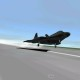# [GSoC2018|OrbitDeterminator|Arya] Week #7-8: Implementing Cowell propagator

Last time when we tried to use the SGP4 propagator in our Kalman Filter, we ran into problems. This was because you can’t stop the algorithm at a certain stage and continue from there. Suppose you want to propagate from to , you can directly propagate from to but you can’t propagate it from to to . So I set out to make our own propagator taking into account two major perturbations – oblateness of Earth and atmospheric drag. Instead of making an analytical propagator like SGP4, I went with a numerical one because that can be stopped and started at any point. So the first challenge was making a numerical integrator.

### Numerical Integration

Numerical integration are used to integrate a differential equation from one point to another point. I studied and tested various integrators and finally settled on Runge-Kutta 4th order integrator (RK4).

• Good accuracy for small to medium step sizes
• Low computation cost
• Easy to implement

Let the initial value problem be and .

Choose a step size and define

for  where,

Note that these equations work equally well whether y is a scalar or a vector. In our case, the differential equation is . It is not in a form suitable to be used directly in RK4. To use it in RK4 we have to vectorize the equation. First, let’s define the state of the system as . Let . Then,This form can be directly used in the RK4 integrator.

### Modelling oblateness of the Earth

Spherical harmonics is used to model the gravity field around the Earth. The method is similar to how Taylor series works. Just like Taylor series, there are various coefficients for each degree of a term in the expansion. The most important coefficient is J2. The next non-zero coefficient J4 is 1000 times smaller than J2. Considering only J2, the gravitational potential around Earth is:

where,
is the radius from the centre
is the latitude of the point with respect to the equator
is a dimensionless constant equal to
is the equatorial radius of the Earth, equal to

To get the acceleration in xyz axes, find the gradient of U and transform coordinates to cartesian. Doing this, we get:

These equations implement J2 perturbation. The main effect of J2 perturbation is precession of the nodes and the argument of perigee.

### Adding drag to the propagator

The simplest model for drag force is . We have to determine the air density, relative velocity and drag coefficients. For air density I used the model given on this website and adjusted the constants for the current solar cycle. The final air density model is:

where h is the altitude of the satellite above the surface of the Earth.

To find the relative velocity, one must account for the rotation of the Earth. where .

After putting together all this, I adjusted the drag coefficient so that satellites decay at approximately the current rate of decay. This finishes the implementation of drag perturbation.

Combining both the perturbations we get:
Putting it in the RK4 integrator completes the propagator.

### Problems faced

I tried the algorithm on a recent TLE of the ISS (shown below).

```ISS (ZARYA)
1 25544U 98067A   18190.66798432  .00001104  00000-0  24004-4 0  9994
2 25544  51.6418 266.6543 0003456 290.0933 212.4518 15.54021918122018```

According to this, it makes 15.54021 revolutions a day. This translates to a time period of 5559.8 secs. However, according to other simulators I found online, the actual period is around 5556 secs. A difference of 4 secs might not sound like much, but over the course of a day it adds up. The Wikipedia page of the ISS lists the time period to be 92.49 mins (5549.4 secs) but also states that it makes 15.54 orbits in a day (5559.8 secs). This is a contradiction. I searched for this issue and tried to correct it, but failed. Right now, the only method is to adjust the semi-major axis by a few kilometres to fix the time period. I will try to correct it later.

### Future Work

• Implementing the Kalman Filter
• Testing against real world satellites

### References## Autor: Arya Das

Computer science student, I like to code, fly planes, and learn about our beautiful universe.

Veröffentlicht am